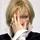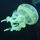## Social Question# Is this a good mathematical explanation?

Asked by LostInParadise (27569) 1 month ago

It seems to me that mathematical explanations and proofs should, whenever possible, relate to our intuition. Here is an example of what I mean. It is a little long, but I hope it is easy to follow.

Suppose we want to find a formula for the sum of the numbers from 1 to n. Let’s consider n=5 and write the numbers: 1 2 3 4 5.

Now let’s ask a simpler question. What would you guess is the average of those numbers? I am hoping that, without much hesitation, you would say 3, the one in the middle. From the average, it is easy to get the sum. We just multiply by 5 to get 15, and adding the numbers we do indeed get 15.

Let’s suppose for now that we can generalize this to all numbers n. How do we find the value in the middle without writing down all the numbers? That is easy. It is the average of the first and the last, (n+1)/2. We therefore get the sum by multiplying by n to get n(n+1)/2.

Let’s now use what we have found to come up with a formal derivation. We note that not only do the first and last add up to n+1, but so the the second and next to last (2 and n-1), the third and third to last (3 and n-2), and so on. Each time one number goes up by 1 in value and the other goes down by 1. If we write the numbers 1 to n and underneath write the numbers from n down to 1, we get n pairs of numbers adding to n+1. Therefore twice the sum of the numbers is n(n+1) and the sum must be half of that, or n(n+1)/2.

Observing members: 0Composing members: 0But the one-in-the-middle solution works only when there’s an odd number of integers, and the n+1 solution only when there’s an even number, isn’t that so?

I think it’s nice when intuition leads us in the same direction as logic, but it can mislead us too, as Mlodinow so effectively established in The Drunkard’s Walk: How Randomness Rules Our Lives. When they part company, logic has to win; it can’t be constrained by a hunch.

Jeruba (50168)“Great Answer” (2) Flag as…We are talking about average, which does not have to be a whole number. Both (n+1)/2 and one in the middle clearly work for odd numbers. Perhaps I should have given an example for an even number. If we write 1, 2, 3, 4, the middle value is between 2 and 3 or 2½, which equals (4+1)/2. There is no need for the average to be one of the numbers from 1 to n.

I agree that intuition can be misleading, which is why I included a formal proof. Intuition can lead to proof, but is not a replacement for it.

LostInParadise (27569)“Great Answer” (1) Flag as…I understand “average.” It was your suggestion of finding the average by choosing the integer in the middle that led to my question.

Jeruba (50168)“Great Answer” (1) Flag as…Perhaps I should have placed the numbers on a number line. That would suggest that the value in the middle need not be a whole number.

LostInParadise (27569)“Great Answer” (2) Flag as…Your explanation of the sum of the first n natural numbers describes in words the mathematical proof therefore it is good. Give yourself a nice big quod erat demonstrandum.

doyendroll (410)“Great Answer” (1) Flag as…